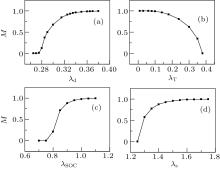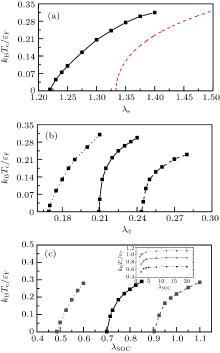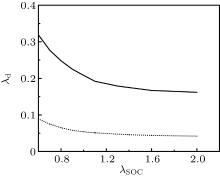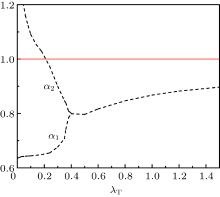Chin. Phys. B, 2020, Vol. 29(11): 110306    DOI: 10.1088/1674-1056/aba9ca
 RAPID COMMUNICATION Prev   Next

# Ferromagnetic transition of a spin–orbit coupled dipolar Fermi gas at finite temperature

Xue-Jing Feng(冯雪景) and Lan Yin(尹澜)
School of Physics, Peking University, Beijing 100871, China
 Abstract  We study the ferromagnetic transition of a two-component homogeneous dipolar Fermi gas with 1D spin–orbit coupling (SOC) at finite temperature. The ferromagnetic transition temperature is obtained as functions of dipolar constant λd, spin–orbit coupling constant λSOC and contact interaction constant λs. It increases monotonically with these three parameters. In the ferromagnetic phase, the Fermi surfaces of different components can be deformed differently. The phase diagrams at finite temperature are obtained. Keywords:  dipolar Fermi gas      stoner model      spin-orbit coupling Received:  15 May 2020      Revised:  29 June 2020      Accepted manuscript online:  28 July 2020 Fund: the National Key Research and Development Project of China (Grant No. 2016YFA0301501). Corresponding Authors:  †Corresponding author. E-mail: yinlan@pku.edu.cnFig. 1.  Magnetization M as functions of λd, λT, λSOC, and λs. (a) M increases with λd for λSOC = 0.7, λT = 0.1 and λs = 0; (b) M decreases with λT for λSOC = 1, λd = 0.26 and λs = 0; (c) M increases with λSOC for λd = 0.25, λT = 0.1 and λs = 0; (d) M increases with λs for λSOC, λd = 0.02 and λT = 0.1.Fig. 2.  Ferromagnetic transition temperature as functions of λs, λd, λSOC. In (a), the solid line is the ferromagnetic transition temperature as a function of λs with λSOC = 0.6 and λd = 0.02 and the red dashed line is the result of the Stoner model. In (b), the solid line is the temperature for λSOC = 1 and λs = 0. The dashed line is the temperature for λSOC = 0.8 and λs = 0. The dotted line is the temperature for λSOC = 1.0 and λs = 0.2. In (c), the solid line is the temperature for λd = 0.27 and λs = 0. The dashed line is the temperature for λd = 0.22 and λs = 0. The dotted line is the temperature for λd = 0.27 and λs = 0.4. In the inset, these lines are saturated to different values at large λSOC.Fig. 3.  Phase diagram at λT = 0.1. The horizontal axis represents SOC constant λSOC and the vertical axis represents DDI constant λd. The solid and dotted lines are ferromagnetic transition lines. The solid line is for λs = 0 while the dotted line is for λs = 1.Fig. 4.  Parameters α1 and α2 as a function of λT with λSOC = 1.0, λd = 0.26 and λs = 0. As λT increases, α1 and α2 meet at the ferromagnetic transition temperature and then slowly approach to 1 from below, which indicates that the two Fermi surfaces are deformed differently in the ferromagnetic phase. The red solid line stands for the ideal Fermi surface.